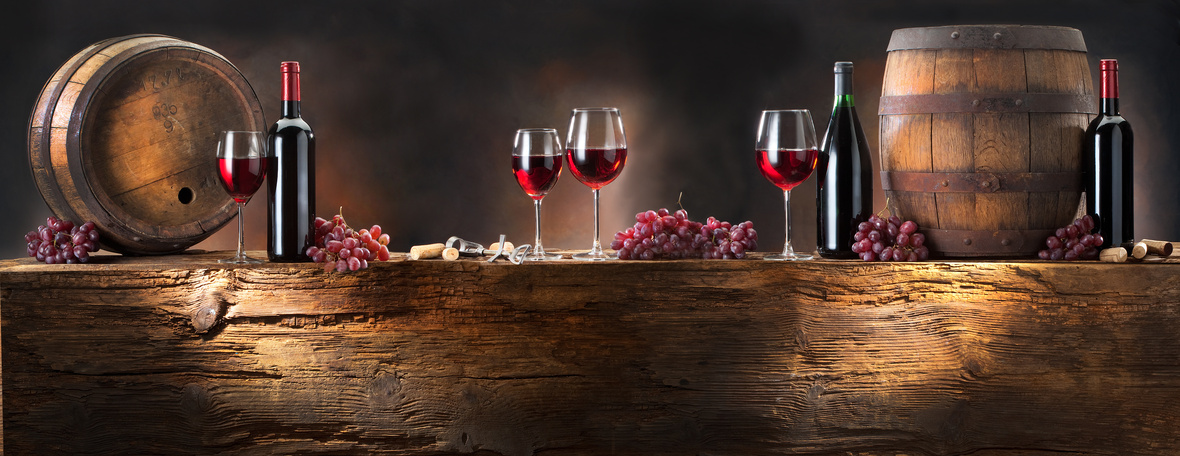# This Just In—New Obsessions and Champagne Style

 /* styles */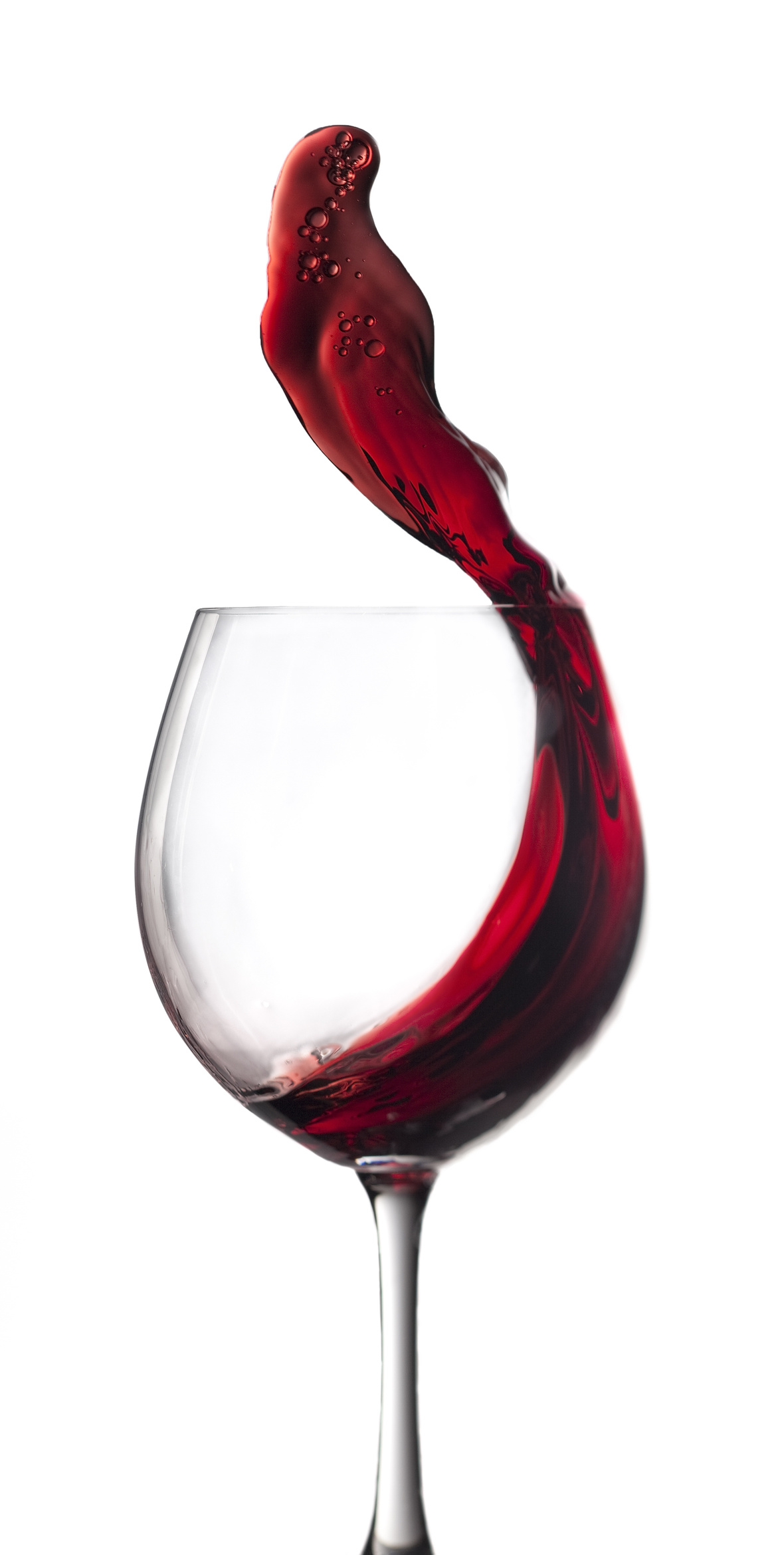Hi Wine & Spirits Lover,

The Shall We Wine team is excited to share our monthly newsletter. Our goal is to introduce you to our favorite selections, producers, distillers, varietals, regions and places to purchase and drink fabulous wine and spirits!

Cheers,

The Shall We Wine Team

 /* styles */

## LIVE IT UP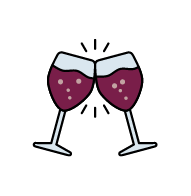Sometimes it’s the small pleasures and little luxuries which give way to a fab-forward, spring season of recharging! This month, Shall We Wine highlights for you distinctive tastes & reads to help put some dazzle in your next pour... whether it's for a grand celebration or a Friday night in.

 table div table+table+table+table+table+table+table+table div table{width:100%;padding:0}table div table+table+table+table+table+table+table+table div table img{width:96.23%;padding:0;float:none}table div table+table+table+table+table+table+table+table div table td{width:100%;padding:0 1.88% 18px}/* styles */## 5 QUESTIONS WITH SHEILA DONOHUE OF VERO

 /* styles */ Shall We Wine caught up with Sheila Donohue—Founder, CEO, and Sommelier of Vero. Vero specializes in limited edition, sustainably-made wines, olive oils and food from around the world! Sheila breaks down for us the mission and inspiration behind Vero and their amazing community: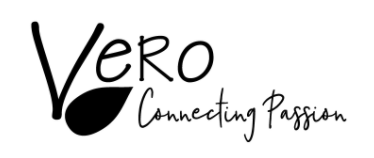/* styles */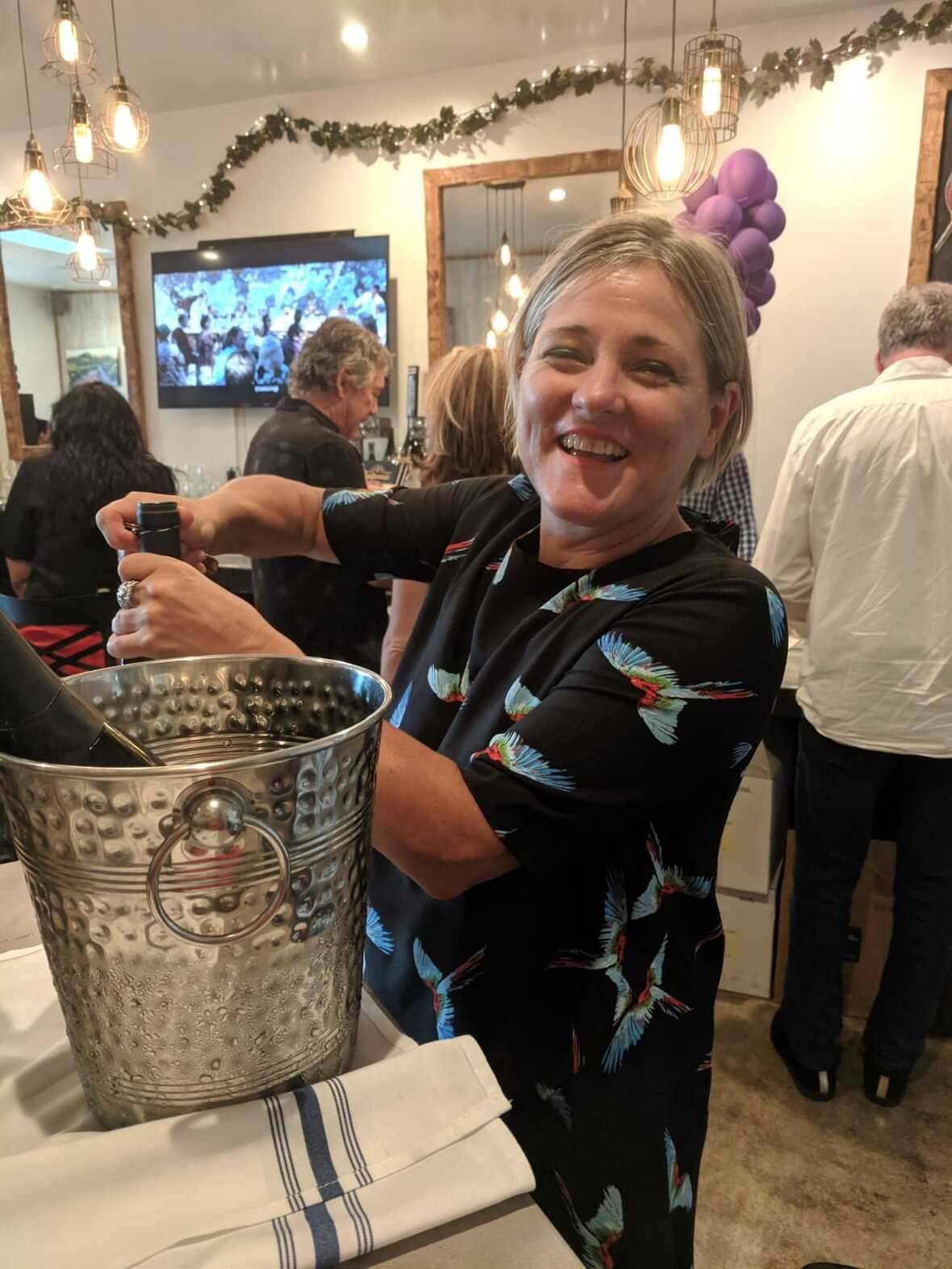1. What is Vero and what is your mission?

Vero seeks out sustainably made wines and olive oils from small producers whose products are missing or hard-to-find in the US market, and we sell them to businesses and consumers across the US. We make it easier for wine and food explorers seeking new flavors and experiences to discover and enjoy craft wine & foods, and connect with the people and stories behind them.

2. What inspired you to start Vero?

I’m a New Yorker who has been in Italy since 2001, working in the banking & technology space. While living in Italy, I’ve met countless passionate artisans who make a living from their land and their craft making delicious products with rich history, traditions and stories behind them. This inspired me to study and become a certified sommelier.

I then had a number of changes in my life, personally and professionally, that left me in 2017 with a year of sabbatical to plan out a new life. Wanting to do something entrepreneurial and innovative, I started Vero in 2018, to open this category of normally inaccessible artisanal products and to become a bridge between Americans and these authentic wines, foods and people.

3. What do the brands you represent have in common?

Our slogan is ‘connecting passion.’ Yeah, our producers are making a livelihood from their wines and foods but passion is what drives them. This same passion is what connects our customers to explore, enjoy and support these small businesses.

Other things our producers have in common:

They are farming their own land, often passed from generation to generation. As a result, they have a love and respect for their territory which drives them to embrace sustainability.

They drink and eat the products they make. They are not market driven but instead driven by tradition and making an authentic product.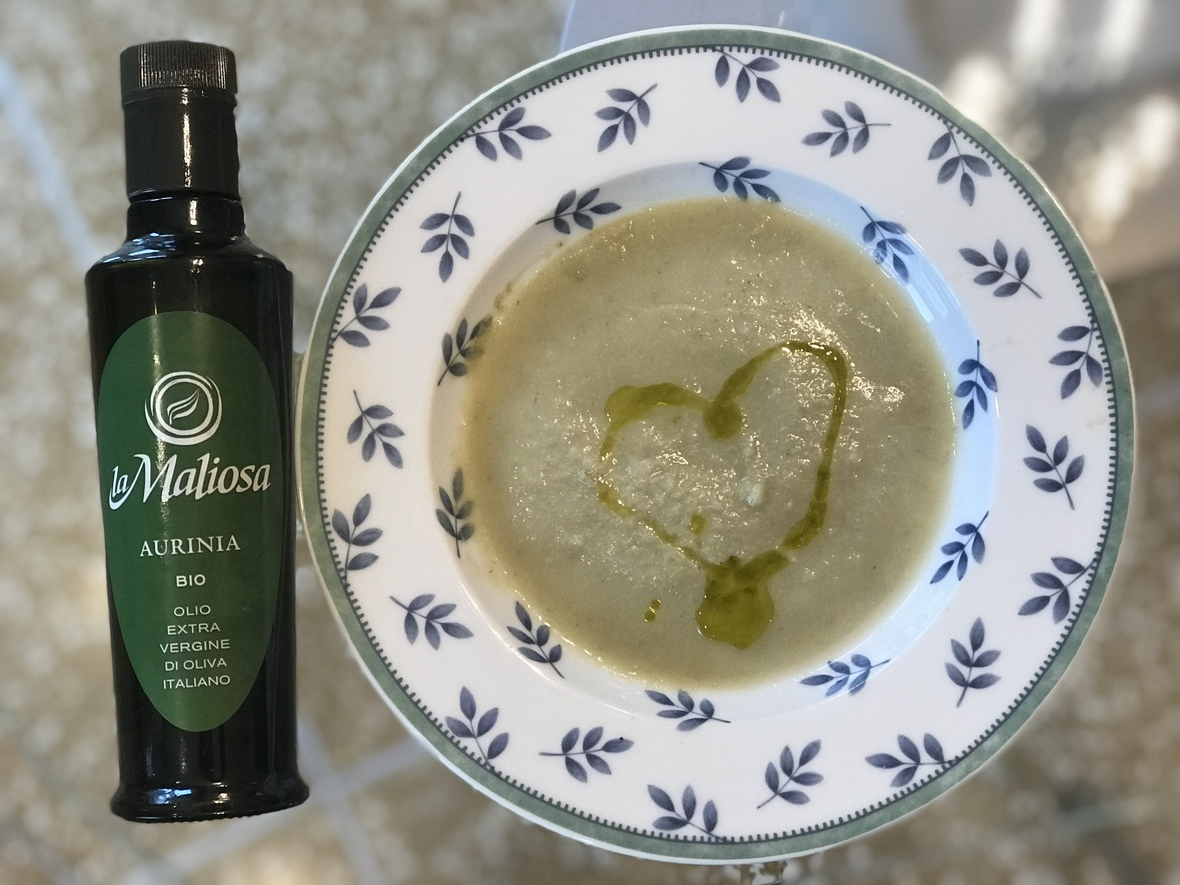4. What does Vero offer its customers?

We have a growing portfolio of craft wines and olive oils from Italy, Spain, CA and soon Portugal too. Before Vero, the products we sell were undiscovered, and for the most part, not available in the US.

Since our business is omnichannel, our customers are across the board, from distributors, wine stores and restaurants to consumers and corporates. Our customers buy from us because they seek something out-of-the-ordinary: not just a beverage, but a product which has a lot of dimensions to it, thus making it more special and enjoyable.

In addition to our selling products, we put on virtual wine and food discovery events, many which involve the producer live from their farm/winery. We also offer private virtual tastings for consumers and corporates.

Our website is www.verovinogusto.com which has an online shop for consumers and corporates to buy directly online. We recently launched a wine club, to put your wine discovery on auto-pilot, with monthly shipments of 3 wines for \$99 shipping included. Sign up for our newsletter to get updates on events, learn about our producers, products, get recipes and have fun exploring!

5. How can Shall We Wine followers find you?

BONUS: Join us Friday, April 30 for a virtual tasting of Vero wines and olive oils. Here is the link to register for the event and purchase the wines we will be tasting at a discount! USE CODE VEROSHALLWEWINE when ordering your wines to receive a discount.

 table div table+table+table+table+table+table+table+table+table+table+table+table+table+table+table div table{width:100%;padding:0}table div table+table+table+table+table+table+table+table+table+table+table+table+table+table+table div table img{width:96.23%;padding:0;float:none}table div table+table+table+table+table+table+table+table+table+table+table+table+table+table+table div table td{width:100%;padding:0 1.88% 18px}/* styles */## DRINK THIS: BILLECART-SALMONElevate any Champagne stockpile with Billecart-Salmon, one of the world's most premier and prestigious Champagne houses.

Calling yourself a historic, family-owned business is easy... but they have receipts! Founded in 1818, this fantastic Champagne house is renowned for exceptional handcrafted quality and excellence. Billecart-Salmon isn't just a trending name. For over 200 years they have been in the biz, now in its 7th generation of running, developing a precise and unique style, and are acclaimed for exquisite Champagne.

 /* styles */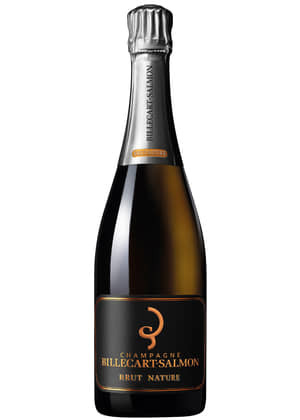Billecart-Salmon Brut Nature Champagne NV

Dry style, with an abundance of elegance and charm! Ages longer on lees in the cellar, aiding in marvelous gravity and complexity.

Graceful. Zero dosage. Enjoy with shrimp, scallops or oysters.

 /* styles */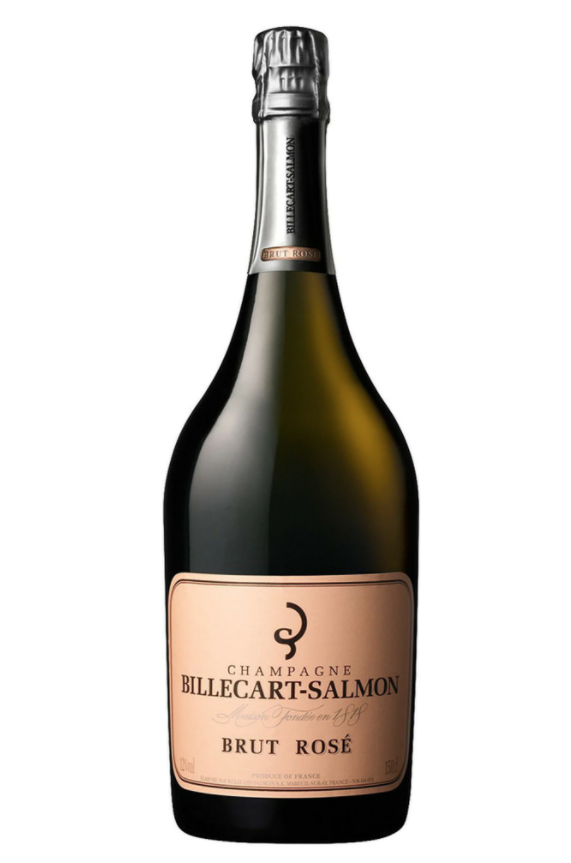Billecart-Salmon Champagne Brut Rosé NV

Billecart-Salmon's flagship cuvée. Bright, fresh character with an expressive, fine mousse. Think strawberries! Vinification in stainless steel. Chardonnay, Pinot Meunier and Pinot Noir vinified as red wine. Amazing pale pink, coral color.

Beautiful. Enjoy with sushi or as an aperitif.

 /* styles */
 table div table+table+table+table+table+table+table+table+table+table+table+table+table+table+table+table+table+table+table+table+table+table+table+table div table{width:100%;padding:0}table div table+table+table+table+table+table+table+table+table+table+table+table+table+table+table+table+table+table+table+table+table+table+table+table div table img{width:96.23%;padding:0;float:none}table div table+table+table+table+table+table+table+table+table+table+table+table+table+table+table+table+table+table+table+table+table+table+table+table div table td{width:100%;padding:0 1.88% 18px}/* styles */## SHALL WE WINE SIPS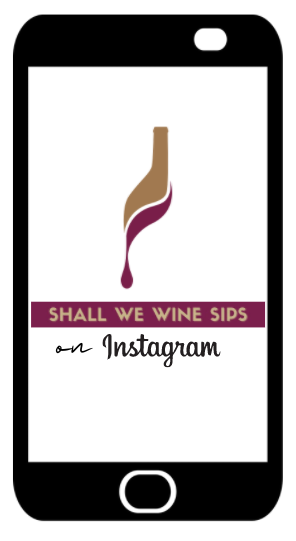/* styles */ Are you following Shall We Wine on IG? Check out our weekly series, SHALL WE WINE SIPS Tuesdays at 6:00 PM CST where we interview some of our fave people in food and wine!
 table div table+table+table+table+table+table+table+table+table+table+table+table+table+table+table+table+table+table+table+table+table+table+table+table+table+table+table+table div table{width:100%;padding:0}table div table+table+table+table+table+table+table+table+table+table+table+table+table+table+table+table+table+table+table+table+table+table+table+table+table+table+table+table div table img{width:96.23%;padding:0;float:none}table div table+table+table+table+table+table+table+table+table+table+table+table+table+table+table+table+table+table+table+table+table+table+table+table+table+table+table+table div table td{width:100%;padding:0 1.88% 18px}/* styles */## 2021 WINE EDUCATION SERIES WITH SWW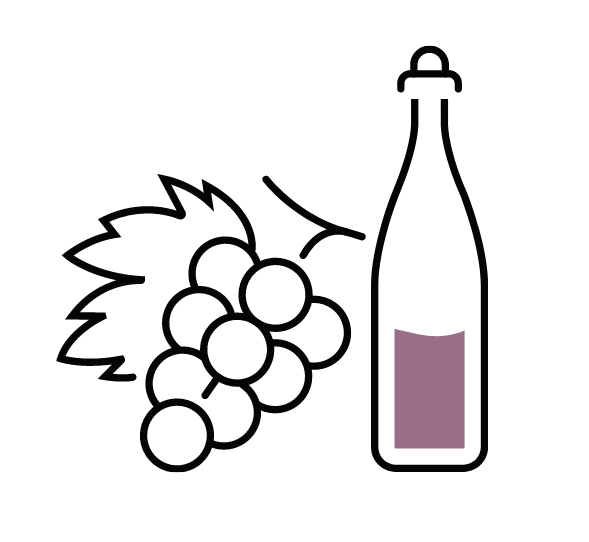Join Regine T. Rousseau, Founder and CEO for more of the amazing line-up of educational events in 2021!

See the list below, and click to reserve your spot.

Want to level up your wine drinking style without the extra fluff? Shall We Wine is a resource that helps translate complex terminology into language that individuals of every level of wine & spirits proficiency can appreciate… all while having fun!

The goal of these classes is to empower you to find the wines that fit your palate, the foods you enjoy and your mood. If you enjoy wine and are looking to deepen your knowledge of wine styles, grapes and regions, these are the classes for you!

 table div table+table+table+table+table+table+table+table+table+table+table+table+table+table+table+table+table+table+table+table+table+table+table+table+table+table+table+table+table+table+table div table{width:100%;padding:0}table div table+table+table+table+table+table+table+table+table+table+table+table+table+table+table+table+table+table+table+table+table+table+table+table+table+table+table+table+table+table+table div table img{width:96.23%;padding:0;float:none}table div table+table+table+table+table+table+table+table+table+table+table+table+table+table+table+table+table+table+table+table+table+table+table+table+table+table+table+table+table+table+table div table td{width:100%;padding:0 1.88% 18px}/* styles *//* styles */table div table+table+table+table+table+table+table+table+table+table+table+table+table+table+table+table+table+table+table+table+table+table+table+table+table+table+table+table+table+table+table+table+table+table div table{width:100%;padding:0}table div table+table+table+table+table+table+table+table+table+table+table+table+table+table+table+table+table+table+table+table+table+table+table+table+table+table+table+table+table+table+table+table+table+table div table img{width:96.23%;padding:0;float:none}table div table+table+table+table+table+table+table+table+table+table+table+table+table+table+table+table+table+table+table+table+table+table+table+table+table+table+table+table+table+table+table+table+table+table div table td{width:100%;padding:0 1.88% 18px}/* styles */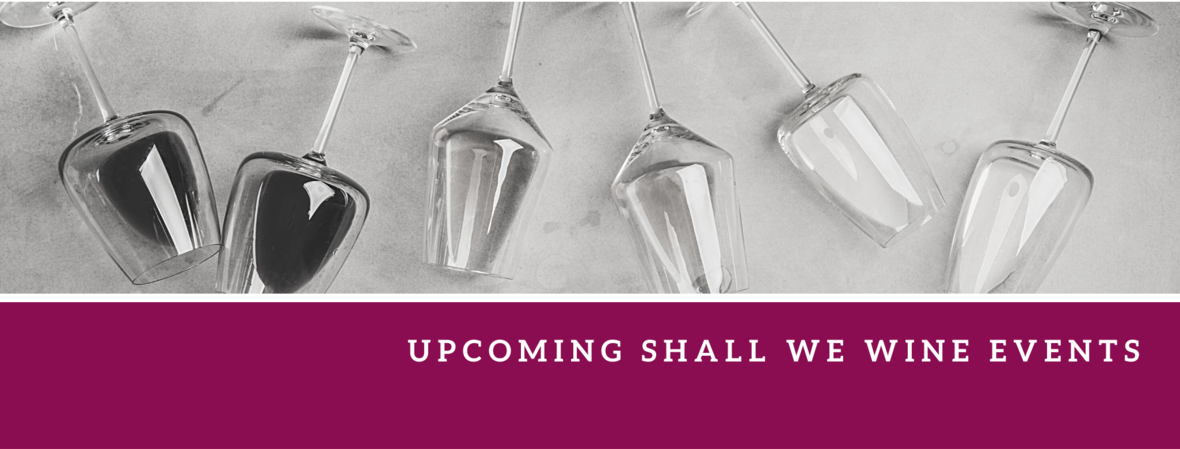/* styles */ Check out our complete events calendar to see what your next pour should be!table div table+table+table+table+table+table+table+table+table+table+table+table+table+table+table+table+table+table+table+table+table+table+table+table+table+table+table+table+table+table+table+table+table+table+table+table+table+table+table+table div table{width:100%;padding:0}table div table+table+table+table+table+table+table+table+table+table+table+table+table+table+table+table+table+table+table+table+table+table+table+table+table+table+table+table+table+table+table+table+table+table+table+table+table+table+table+table div table img{width:96.23%;padding:0;float:none}table div table+table+table+table+table+table+table+table+table+table+table+table+table+table+table+table+table+table+table+table+table+table+table+table+table+table+table+table+table+table+table+table+table+table+table+table+table+table+table+table div table td{width:100%;padding:0 1.88% 18px}/* styles */## NEW OBSESSIONS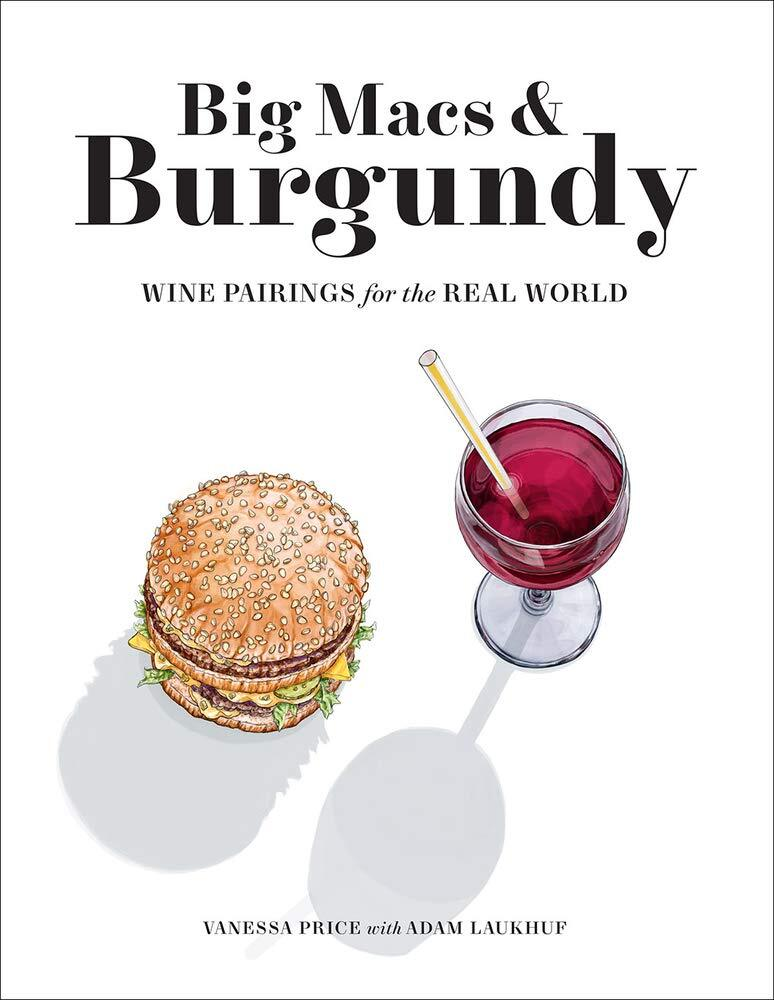Big Macs & Burgundy | Wine Pairings for the Real World
National Best Seller

Love this book! Authors Vanessa Price and Adam Laukhuf create over 300 food and wine adventures!

If you want to learn more about food and wine pairing, or have fun playing with your food, this is the book for you. Purchase the book here.

Regine also had the opportunity to interview Vanessa on IG.

 /* styles */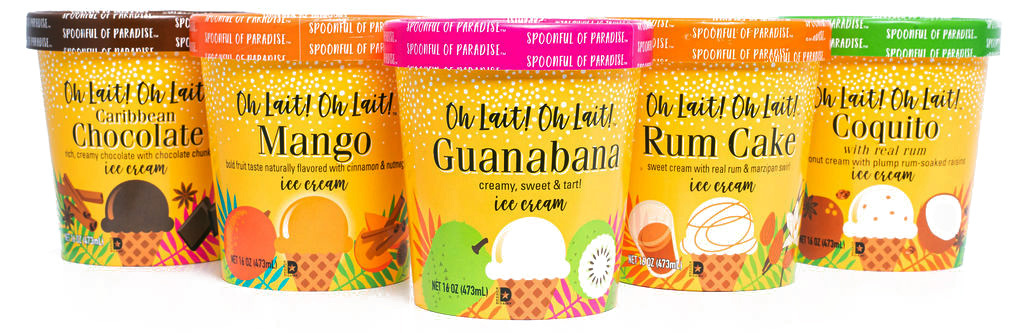Oh Lait! Oh Lait! | Spoonful of Paradise

Oh Lait Oh Lait, a spoonful of paradise!

This caught my attention. These Caribbean-inspired ice creams are decadent, fresh and flavorful! I tasted the OH LAIT! OH LAIT! VARIETY 5 PACK and loved them all. As a Caribbean girl myself, I was quickly transformed to my childhood.

Should be fun to pair these with wines! The Mango or Guanabana can be paired with a Moscato d’Asti and the Caribbean Chocolate can be paired with a 10 year old Tawny Port. Both Rum Cake and Coquito flavors are made with rum, so no need to pair those with wine, unless you just want to! I’m not judging. Order yours today here.

 table div table+table+table+table+table+table+table+table+table+table+table+table+table+table+table+table+table+table+table+table+table+table+table+table+table+table+table+table+table+table+table+table+table+table+table+table+table+table+table+table+table+table+table+table+table div table{width:100%;padding:0}table div table+table+table+table+table+table+table+table+table+table+table+table+table+table+table+table+table+table+table+table+table+table+table+table+table+table+table+table+table+table+table+table+table+table+table+table+table+table+table+table+table+table+table+table+table div table img{width:96.23%;padding:0;float:none}table div table+table+table+table+table+table+table+table+table+table+table+table+table+table+table+table+table+table+table+table+table+table+table+table+table+table+table+table+table+table+table+table+table+table+table+table+table+table+table+table+table+table+table+table+table div table td{width:100%;padding:0 1.88% 18px}/* styles */## BLOG CENTRALWe have a blog! Here you will find things like our Wine of the Week and other special wine & spirits topics! Stay in the know and read up on some of the cool things we've been sharing at Shall We Wine.

Fun Topics:
The Illustrious House of Champagne Billecart-Salmon
Let's Take A Peak: Ant Moore Wines
7 Steps to Reinvent Your Wine & Spirits Self
Wine Podcasts to Listen to Right Now

 table div table+table+table+table+table+table+table+table+table+table+table+table+table+table+table+table+table+table+table+table+table+table+table+table+table+table+table+table+table+table+table+table+table+table+table+table+table+table+table+table+table+table+table+table+table+table+table+table div table{width:100%;padding:0}table div table+table+table+table+table+table+table+table+table+table+table+table+table+table+table+table+table+table+table+table+table+table+table+table+table+table+table+table+table+table+table+table+table+table+table+table+table+table+table+table+table+table+table+table+table+table+table+table div table img{width:96.23%;padding:0;float:none}table div table+table+table+table+table+table+table+table+table+table+table+table+table+table+table+table+table+table+table+table+table+table+table+table+table+table+table+table+table+table+table+table+table+table+table+table+table+table+table+table+table+table+table+table+table+table+table+table div table td{width:100%;padding:0 1.88% 18px}/* styles */## ENGAGING READS FOR THE MONTH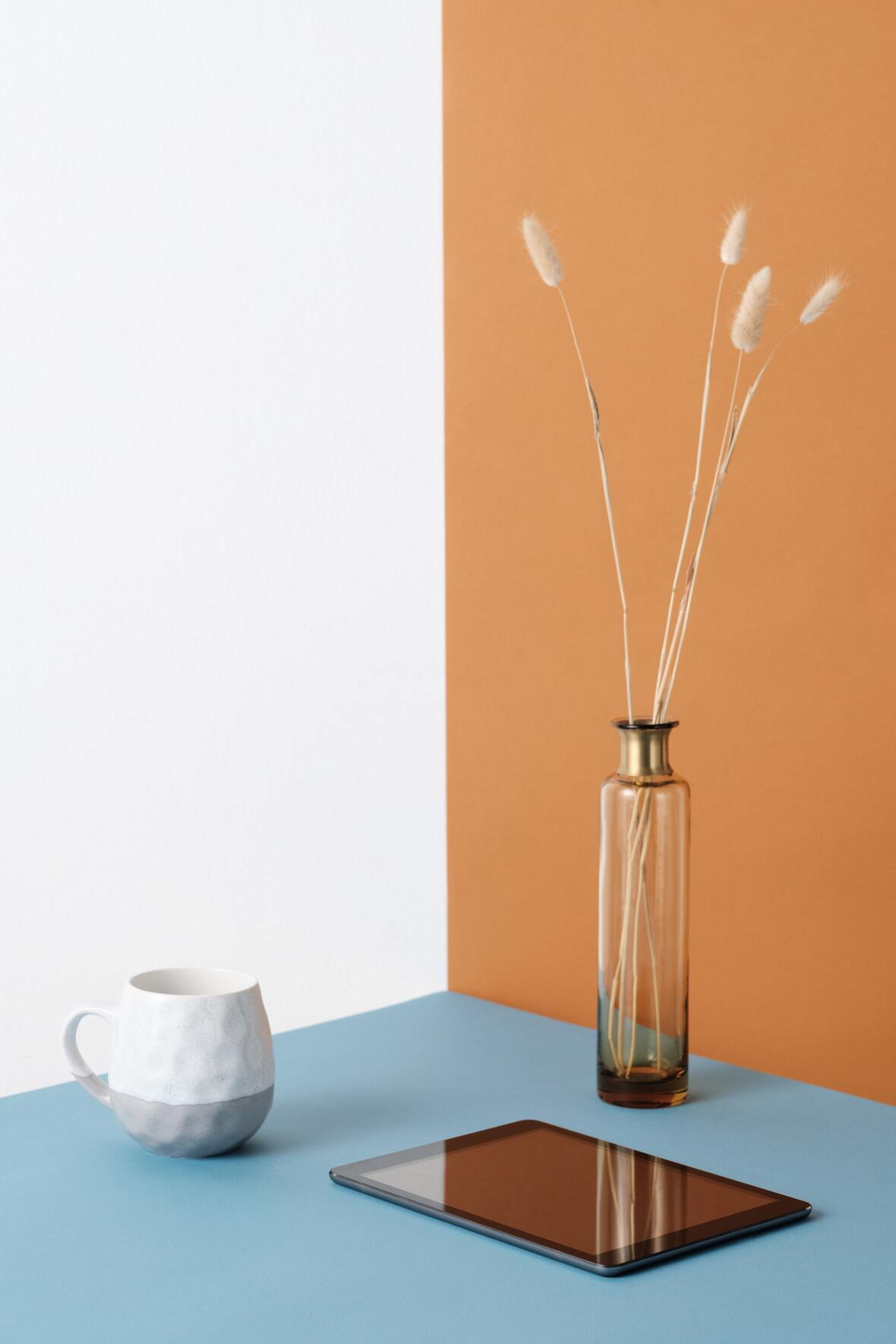Wine Enthusiast

Natural Action Aims to Change Wine World
Santa Barbara Independent

Grenache Three Ways, Over Many Decades
New York Times

 table div table+table+table+table+table+table+table+table+table+table+table+table+table+table+table+table+table+table+table+table+table+table+table+table+table+table+table+table+table+table+table+table+table+table+table+table+table+table+table+table+table+table+table+table+table+table+table+table+table+table+table div table{width:100%;padding:0}table div table+table+table+table+table+table+table+table+table+table+table+table+table+table+table+table+table+table+table+table+table+table+table+table+table+table+table+table+table+table+table+table+table+table+table+table+table+table+table+table+table+table+table+table+table+table+table+table+table+table+table div table img{width:96.23%;padding:0;float:none}table div table+table+table+table+table+table+table+table+table+table+table+table+table+table+table+table+table+table+table+table+table+table+table+table+table+table+table+table+table+table+table+table+table+table+table+table+table+table+table+table+table+table+table+table+table+table+table+table+table+table+table div table td{width:100%;padding:0 1.88% 18px}/* styles */## STAY CONNECTED

 /* styles */ To stay in the know with the latest and greatest, make sure to follow us! Links are below.
 table div table+table+table+table+table+table+table+table+table+table+table+table+table+table+table+table+table+table+table+table+table+table+table+table+table+table+table+table+table+table+table+table+table+table+table+table+table+table+table+table+table+table+table+table+table+table+table+table+table+table+table+table+table+table div table{width:100%;padding:0}table div table+table+table+table+table+table+table+table+table+table+table+table+table+table+table+table+table+table+table+table+table+table+table+table+table+table+table+table+table+table+table+table+table+table+table+table+table+table+table+table+table+table+table+table+table+table+table+table+table+table+table+table+table+table div table table{padding:0;float:left!important;width:26.059%!important}table div table+table+table+table+table+table+table+table+table+table+table+table+table+table+table+table+table+table+table+table+table+table+table+table+table+table+table+table+table+table+table+table+table+table+table+table+table+table+table+table+table+table+table+table+table+table+table+table+table+table+table+table+table+table div table td{padding-left:29px;padding-right:29px}table div table+table+table+table+table+table+table+table+table+table+table+table+table+table+table+table+table+table+table+table+table+table+table+table+table+table+table+table+table+table+table+table+table+table+table+table+table+table+table+table+table+table+table+table+table+table+table+table+table+table+table+table+table+table div table table+table,table div table+table+table+table+table+table+table+table+table+table+table+table+table+table+table+table+table+table+table+table+table+table+table+table+table+table+table+table+table+table+table+table+table+table+table+table+table+table+table+table+table+table+table+table+table+table+table+table+table+table+table+table+table+table div table table+table+table{float:left!important;width:26.059%!important}table div table+table+table+table+table+table+table+table+table+table+table+table+table+table+table+table+table+table+table+table+table+table+table+table+table+table+table+table+table+table+table+table+table+table+table+table+table+table+table+table+table+table+table+table+table+table+table+table+table+table+table+table+table+table div table table td,table div table+table+table+table+table+table+table+table+table+table+table+table+table+table+table+table+table+table+table+table+table+table+table+table+table+table+table+table+table+table+table+table+table+table+table+table+table+table+table+table+table+table+table+table+table+table+table+table+table+table+table+table+table+table div table table+table td,table div table+table+table+table+table+table+table+table+table+table+table+table+table+table+table+table+table+table+table+table+table+table+table+table+table+table+table+table+table+table+table+table+table+table+table+table+table+table+table+table+table+table+table+table+table+table+table+table+table+table+table+table+table+table div table table+table+table td{padding-left:0;padding-right:20px}table div table+table+table+table+table+table+table+table+table+table+table+table+table+table+table+table+table+table+table+table+table+table+table+table+table+table+table+table+table+table+table+table+table+table+table+table+table+table+table+table+table+table+table+table+table+table+table+table+table+table+table+table+table+table div table table+table+table+table{float:left!important;width:21.822999999999993%!important}table div table+table+table+table+table+table+table+table+table+table+table+table+table+table+table+table+table+table+table+table+table+table+table+table+table+table+table+table+table+table+table+table+table+table+table+table+table+table+table+table+table+table+table+table+table+table+table+table+table+table+table+table+table+table div table table+table+table+table td{padding-left:0;padding-right:0}/* styles *//* styles */ Learn, Drink, Repeat! The Shall We Wine Team
 Like   Tweet   Pin   +1   in